# Displaying all data points in a Matplotlib graph¶

I routinely use R to manipulate and plot the data I get from my experiments, but I’m currently exploring the possibilities of IPython, NumPy, and Matplotlib. Here are a few notes after my first steps with these tools.

## Let’s have some data¶

In :
```%matplotlib inline
import matplotlib.pyplot as plt
import numpy as np
```

Then I input some raw data. For this example, I will use actual data from the times of my PhD thesis:

In :
```ctrl = np.array([.91, .88, .94, 1.0, 1.0, 1.0, .85, 1.0, 1.0, .75, 1.0, 1.0, 1.0, .85, 1.0, .91, .81, 1.0, .91, .86])
slcd = np.array([.33, .67, .75, 1.0, .71, .63, .46, .71, .56, .75, .71, .57, .71, .55, .71, .58, .63, .64, .64, .89])
```

A bit of background, just to understand what these values represent: In that experiment, I treated cultured human cells with either a control reagent (“ctrl”) or with a reagent that decreases the level of expression of a given gene (that gene is said to be silenced, hence “slcd”); then, in several cells I counted how many centromeres were associated with the CENP-C protein. Each value above represents one cell, and “0.5”, for example, means that 50% of the centromeres in that cell were occupied by CENP-C. In normal conditions, centromere occupancy by CENP-C is expected to be complete (CENP-C is present on all centromeres); the purpose of the experiment was to determine whether silencing the gene of interest had an effect over centromere occupancy.

Now I compute some summary statistics (after converting all the values to percentages)…

In :
```ctrl *= 100
slcd *= 100
means = [np.mean(ctrl), np.mean(slcd)]
stdvs = [np.std(ctrl), np.std(slcd)]
```

and plot the results in a typical “dynamite plot”-style bar graph:

In :
```plt.figure(figsize=(3,4))
plt.bar([0, 1], means, .5, yerr=stdvs, align='center')
plt.xticks([0,1], ("Control", "Silenced"))
plt.xlabel("Condition")
plt.ylim(0,110)
plt.ylabel("Centromere occupancy (%)")
plt.draw()
```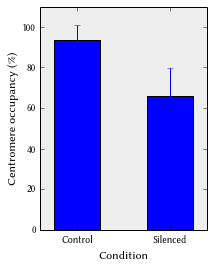## Show the atoms¶

When plotting data, I like to plot all the data instead of only some summary statistics (means, standard errors, and the like). Quoting Rafe Donahue, in his Principles for Constructing Better Graphics, “Each datum gets one glob of ink.”

Unfortunately, this use case is not covered in the (impressive) Matplotlib gallery. There may be several ways of doing that kind of plots, here is what I came up with at my first attempt:

In :
```plt.figure(figsize=(3,4))
plt.plot([0 for v in ctrl], ctrl, '.', color='b')
plt.plot([1 for v in slcd], slcd, '.', color='r')
plt.xlim(-0.5, 1.5)
plt.xticks([0, 1], ("Control", "Silenced"))
plt.xlabel("Condition")
plt.ylim(0,105)
plt.ylabel("Centromere occupancy")
plt.draw()
```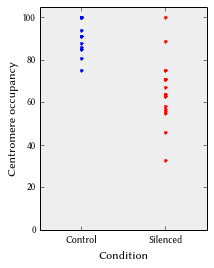An obvious problem with that method is that all points for a given condition are aligned on the same X coordinate. When several points have the same value, they appear as one. This gives a false idea of the actual distribution of data.

I tried to overcome that problem by randomizing the X coordinate of all points:

In :
```plt.figure(figsize=(3,4))
# Randomly puts control points between -0.3 and +0.3
ctrl_x = .6 * np.random.random_sample(size=len(ctrl)) - .3
# Randomly puts silenced points between 0.7 and 1.3
slcd_x = .6 * np.random.random_sample(size=len(slcd)) + .7
plt.plot(ctrl_x, ctrl, '.', color='b')
plt.plot(slcd_x, slcd, '.', color='r')
plt.xlim(-.5, 1.5)
plt.xticks([0, 1], ("Control", "Silenced"))
plt.xlabel("Condition")
plt.ylim(0,105)
plt.ylabel("Centromere occupancy (%)")
plt.draw()
```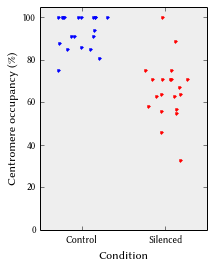That’s better, but I would prefer something less… random. I ended up writing my own function to properly disperse X coordinates:

In :
```def xdistr(values, width, offset=0, ticks=10, center=False):
dist = {}
spent = {}
xcoords = []

for v in values:
if dist.has_key(v):
dist[v] += 1
else:
dist[v] = 1
spent[v] = 0

for v in values:
# Compute the horizontal distance between dots
step = width / max(ticks, dist[v])

if not center:
x = offset + step * spent[v]
else:
# If we've already drawn an even number of dots,
# draw the next one on the right
if spent[v] % 2 == 0:
x = offset + (step / 2) * spent[v]
else:
# otherwise draw the next dot on the left
x = offset - (step / 2) * (spent[v] + 1)

# If there is an even number of dots, shift all
# dots by a half-step to center the rank
if dist[v] % 2 == 0:
x += step / 2

spent[v] += 1
xcoords.append(x)

return xcoords
```

This function can then replace the randomizing step above:

In :
```plt.figure(figsize=(3,4))
ctrl_x = xdistr(ctrl, 0.6, center=True)
slcd_x = xdistr(slcd, 0.6, offset=1, center=True)
plt.plot(ctrl_x, ctrl, '.', color='b')
plt.plot(slcd_x, slcd, '.', color='r')
plt.xlim(-.5, 1.5)
plt.xticks([0, 1], ("Control", "Silenced"))
plt.xlabel("Condition")
plt.ylim(0,105)
plt.ylabel("Centromere occupancy (%)")
plt.draw()
```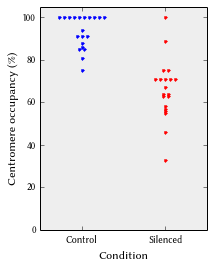Nearly there! A last improvement is to separate some points that have different Y values but are still too close to each other:

In :
```plt.figure(figsize=(3,4))
ctrl_x = xdistr([int(i) for i in ctrl/3], 0.6, center=True)
slcd_x = xdistr([int(i) for i in slcd/3], 0.6, offset=1, center=True)
plt.plot(ctrl_x, ctrl, '.', color='b')
plt.plot(slcd_x, slcd, '.', color='r')
plt.xlim(-.5, 1.5)
plt.xticks([0, 1], ("Control", "Silenced"))
plt.xlabel("Condition")
plt.ylim(0,105)
plt.ylabel("Centromere occupancy (%)")
plt.draw()
```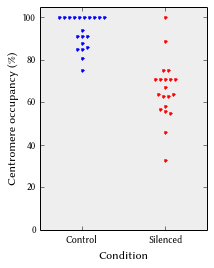And that, finally, is exactly what I wanted.